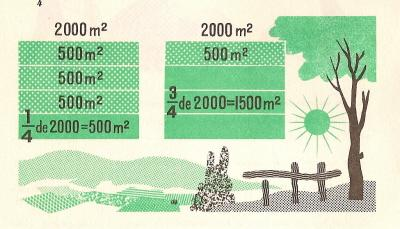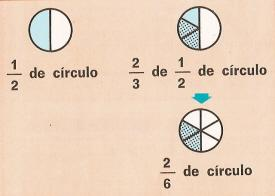Multiplication of fractions to printName: ______________________________________  Subject: _______________________ Date: _______

Write the missing word

1.- Multiplication of a fraction by a number.

A field is 2000 square meters. How many square meters does Ľ and 3/4 of the field have?

We divide the field into four parts and each part (1/4) will be 500 square meters. 3/4 will have 3 parts, that is, 1500 square meters.

1/4 of 2000 = 1/4 x 2000 = (1 x 2000) / 4 = 2000/4 = 500.

3/4 of 2000 = 3/4 x 2000 = (3 x 2000) / 4 = 6000/4 = 1500.

To take a fraction of a number, we multiply it by that number.

To multiply a fraction by a number, we multiply the numerator by that number.

Solve these exercises:

 1/7 de 14 = 3/5 de 500 = 3/8 de 160 = 1/3 de 90 =2.- Multiplication of Fractions

2/3 of 1/2 circle = 2/6. We have multiplied the two fractions: 2/3 x 1/2 = (2x1) / (3x2) = 2/6.

A fraction of a fraction is equal to the product of both fractions.

To multiply two fractions, we multiply the numerators to form the numerator of the product, and then we multiply the denominators to form the denominator of the product.

Examples: 2/3 x 1/4 = (2x1) / (3x4) = 2/12 = 1/6; 4/7 x 3/5 = (4x3) / (7x5) = 12/35, 1/5 × 3/4 = 3/20.

Multiply these fractions:

 1/3 x 2/5 = 2/3 x 1/7 = 3/4 x 1/5 = 1/8 x 4/9 =

3.- Solve these problems:

 1.The first stage in Spain is 253 km. A cyclist has went over 5/11 of the route in 2 hours. How many kilometers does he need to go over to complete the stage? 2. A secretary earns daily 42 1/6 euros and she spends 38 2/6 euros. How much money does she save daily? 3. Ana has Ľ of a cake and Arthur has 1/6. How much pieces of cake do they have in total? 4. We eat 3/7 of a cake and then we eat 1/8. How much do we have to eat to finish the cake? 5. How much cost 157 liters of wine if we sell it to 2/9 euros per liter? 6. A trader sells 2/5 of a ton of coal to 1/7 euros per kilogram. How much euros does he charge? 7. How many minutes is 2/3 of ˝ hour? 8. A man paints a room in 5 hours. What part of the room does he paint in 1 hour?

| Educational applications | Mathematics |
In Spanish
Interactive |

®Arturo Ramo García.-Record of intellectual property of Teruel (Spain) No 141, of 29-IX-1999
Plaza Playa de Aro, 3, 1ş DO 44002-TERUEL